Open in App
Not now

# Logic Gates in Python

• Difficulty Level : Easy
• Last Updated : 11 Apr, 2022

Logic gates are elementary building blocks for any digital circuits. It takes one or two inputs and produces output based on those inputs. Outputs may be high (1) or low (0). Logic gates are implemented using diodes or transistors. It can also be constructed using vacuum tubes, electromagnetic elements like optics, molecules, etc. In a computer, most of the electronic circuits are made up of logic gates. Logic gates are used to circuits that perform calculations, data storage, or show off object-oriented programming especially the power of inheritance.

There are seven basic logic gates defined are: AND gate, OR gate, NOT gate, NAND gate, NOR gate, XOR gate, an XNOR gate.

1. AND Gate
The AND gate gives an output of 1 if both the two inputs are 1, it gives 0 otherwise.## Python3

 `# Python3 program to illustrate``# working of AND gate` `def` `AND (a, b):` `    ``if` `a ``=``=` `1` `and` `b ``=``=` `1``:``        ``return` `True``    ``else``:``        ``return` `False` `# Driver code``if` `__name__``=``=``'__main__'``:``    ``print``(AND(``1``, ``1``))` `    ``print``(``"+---------------+----------------+"``)``    ``print``(``" | AND Truth Table | Result |"``)``    ``print``(``" A = False, B = False | A AND B ="``,AND(``False``,``False``),``" | "``)``    ``print``(``" A = False, B = True | A AND B ="``,AND(``False``,``True``),``" | "``)``    ``print``(``" A = True, B = False | A AND B ="``,AND(``True``,``False``),``" | "``)``    ``print``(``" A = True, B = True | A AND B ="``,AND(``True``,``True``),``" | "``)`

Output:

```True
+---------------+----------------
| AND Truth Table |    Result |
A = False, B = False | A AND B = False  |
A = False, B = True  | A AND B = False  |
A = True, B = False  | A AND B = False  |
A = True, B = True   | A AND B = True   | ```

2. NAND Gate
The NAND gate (negated AND) gives an output of 0 if both inputs are 1, it gives 1 otherwise.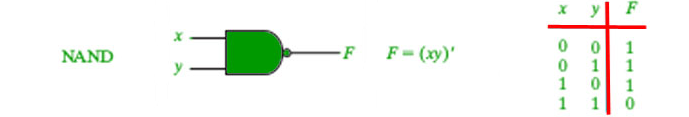## Python3

 `# Python3 program to illustrate``# working of NAND gate` `def` `NAND (a, b):``    ``if` `a ``=``=` `1` `and` `b ``=``=` `1``:``        ``return` `False``    ``else``:``        ``return` `True` `# Driver code``if` `__name__``=``=``'__main__'``:``    ``print``(NAND(``1``, ``0``))` `    ``print``(``"+---------------+----------------+"``)``    ``print``(``" | NAND Truth Table | Result |"``)``    ``print``(``" A = False, B = False | A AND B ="``,NAND(``False``,``False``),``" | "``)``    ``print``(``" A = False, B = True | A AND B ="``,NAND(``False``,``True``),``" | "``)``    ``print``(``" A = True, B = False | A AND B ="``,NAND(``True``,``False``),``" | "``)``    ``print``(``" A = True, B = True | A AND B ="``,NAND(``True``,``True``),``" | "``)`

Output:

```True
+---------------+----------------
| NAND Truth Table |    Result |
A = False, B = False | A AND B = True  |
A = False, B = True  | A AND B = True  |
A = True, B = False  | A AND B = True  |
A = True, B = True   | A AND B = False | ```

3. OR Gate
The OR gate gives an output of 1 if either of the two inputs are 1, it gives 0 otherwise.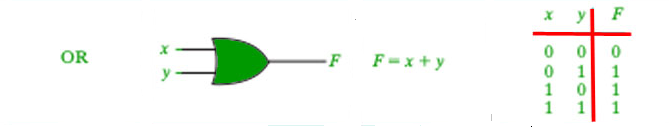## Python3

 `# Python3 program to illustrate``# working of OR gate` `def` `OR(a, b):``    ``if` `a ``=``=` `1` `or` `b ``=``=``1``:``        ``return` `True``    ``else``:``        ``return` `False` `# Driver code``if` `__name__``=``=``'__main__'``:``    ``print``(OR(``0``, ``0``))` `    ``print``(``"+---------------+----------------+"``)``    ``print``(``" | OR Truth Table | Result |"``)``    ``print``(``" A = False, B = False | A OR B ="``,OR(``False``,``False``),``" | "``)``    ``print``(``" A = False, B = True | A OR B ="``,OR(``False``,``True``),``" | "``)``    ``print``(``" A = True, B = False | A OR B ="``,OR(``True``,``False``),``" | "``)``    ``print``(``" A = True, B = True | A OR B ="``,OR(``True``,``True``),``" | "``)`

Output:

```False
+---------------+----------------+
| OR Truth Table |    Result |
A = False, B = False | A OR B = False  |
A = False, B = True  | A OR B = True   |
A = True, B = False  | A OR B = True   |
A = True, B = True   | A OR B = True   | ```

4. XOR Gate
The XOR gate gives an output of 1 if either of the inputs is different, it gives 0 if they are the same.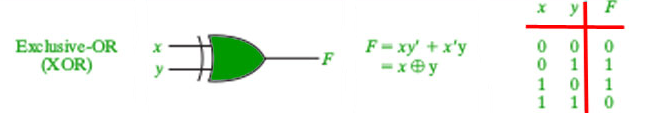## Python3

 `# Python3 program to illustrate``# working of Xor gate` `def` `XOR (a, b):``    ``if` `a !``=` `b:``        ``return` `1``    ``else``:``        ``return` `0` `# Driver code``if` `__name__``=``=``'__main__'``:``    ``print``(XOR(``5``, ``5``))` `    ``print``(``"+---------------+----------------+"``)``    ``print``(``" | XOR Truth Table | Result |"``)``    ``print``(``" A = False, B = False | A XOR B ="``,XOR(``False``,``False``),``" | "``)``    ``print``(``" A = False, B = True | A XOR B ="``,XOR(``False``,``True``),``" | "``)``    ``print``(``" A = True, B = False | A XOR B ="``,XOR(``True``,``False``),``" | "``)``    ``print``(``" A = True, B = True | A XOR B ="``,XOR(``True``,``True``),``" | "``)`

Output:

```0
+---------------+----------------+
| XOR Truth Table | Result |
A = False, B = False | A XOR B = 0  |
A = False, B = True  | A XOR B = 1  |
A = True, B = False  | A XOR B = 1  |
A = True, B = True   | A XOR B = 0  | ```

5. NOT Gate
It acts as an inverter. It takes only one input. If the input is given as 1, it will invert the result as 0 and vice-versa.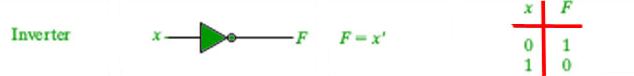## Python3

 `# Python3 program to illustrate``# working of Not gate` `def` `NOT(a):``    ``return` `not` `a``# Driver code``if` `__name__``=``=``'__main__'``:``    ``print``(NOT(``0``))` `    ``print``(``"+---------------+----------------+"``)``    ``print``(``" | NOT Truth Table | Result |"``)``    ``print``(``" A = False | A NOT ="``,NOT(``False``),``" | "``)``    ``print``(``" A = True, | A NOT ="``,NOT(``True``),``" | "``)`` `

Output:

```1
+---------------+----------------+
| NOT Truth Table | Result |
A = False | A NOT = 1  |
A = True, | A NOT = 0  | ```

6. NOR Gate
The NOR gate (negated OR) gives an output of 1 if both inputs are 0, it gives 0 otherwise.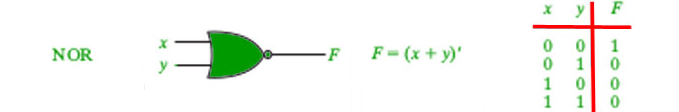## Python3

 `# Python3 program to illustrate``# working of NOR gate` `def` `NOR(a, b):``    ``if``(a ``=``=` `0``) ``and` `(b ``=``=` `0``):``        ``return` `1``    ``elif``(a ``=``=` `0``) ``and` `(b ``=``=` `1``):``        ``return` `0``    ``elif``(a ``=``=` `1``) ``and` `(b ``=``=` `0``):``        ``return` `0``    ``elif``(a ``=``=` `1``) ``and` `(b ``=``=` `1``):``        ``return` `0` `# Driver code``if` `__name__``=``=``'__main__'``:``    ``print``(NOR(``0``, ``0``))` `    ``print``(``"+---------------+----------------+"``)``    ``print``(``" | NOR Truth Table | Result |"``)``    ``print``(``" A = False, B = False | A NOR B ="``,NOR(``False``,``False``),``" | "``)``    ``print``(``" A = False, B = True | A NOR B ="``,NOR(``False``,``True``),``" | "``)``    ``print``(``" A = True, B = False | A NOR B ="``,NOR(``True``,``False``),``" | "``)``    ``print``(``" A = True, B = True | A NOR B ="``,NOR(``True``,``True``),``" | "``)`

Output:

```1
+---------------+----------------+
| NOR Truth Table |   Result |
A = False, B = False | A NOR B = 1  |
A = False, B = True  | A NOR B = 0  |
A = True, B = False  | A NOR B = 0  |
A = True, B = True   | A NOR B = 0  | ```

7. XNOR Gate
The XNOR gate (negated XOR) gives an output of 1 both inputs are same and 0 if both are different.## Python3

 `# Python3 program to illustrate``# working of Not gate` `def` `XNOR(a,b):``    ``if``(a ``=``=` `b):``        ``return` `1``    ``else``:``        ``return` `0``# Driver code``if` `__name__``=``=``'__main__'``:``    ``print``(XNOR(``1``,``1``))` `    ``print``(``"+---------------+----------------+"``)``    ``print``(``" | XNOR Truth Table | Result |"``)``    ``print``(``" A = False, B = False | A XNOR B ="``,XNOR(``False``,``False``),``" | "``)``    ``print``(``" A = False, B = True | A XNOR B ="``,XNOR(``False``,``True``),``" | "``)``    ``print``(``" A = True, B = False | A XNOR B ="``,XNOR(``True``,``False``),``" | "``)``    ``print``(``" A = True, B = True | A XNOR B ="``,XNOR(``True``,``True``),``" | "``)`

Output:

```1
+---------------+----------------+
| XNOR Truth Table |  Result |
A = False, B = False | A XNOR B = 1  |
A = False, B = True  | A XNOR B = 0  |
A = True, B = False  | A XNOR B = 0  |
A = True, B = True   | A XNOR B = 1  | ```

My Personal Notes arrow_drop_up